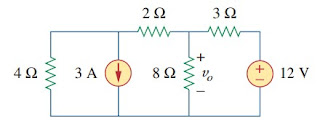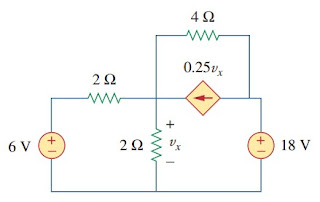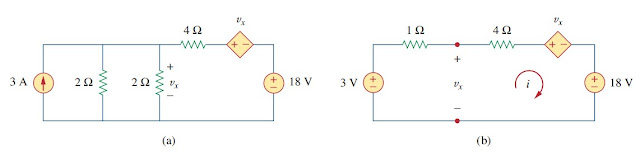omG8HI9LSKFW6lhCj8prTs0Z6lUhdOT9Jhi1Sf4m

# Source Transformation

We have noticed that series-parallel combination and wye-delta transformation help simplify circuits. Source transformation is another tool for simplifying circuits. Basic to these tools is the concept of equivalence. We recall that an equivalent circuit is one whose v-i characteristics are identical with the original circuit.

This circuit analysis theorems are classified as:
1. Superposition theorem
2. Source transformation
3. Thevenin theorem
4. Norton theorem
5. Maximum power transfer

## Source Transformation

In previous posts about node voltage or mesh current equations can be obtained by mere inspection of a circuit when the sources are all independent current or independent voltage sources. It is therefore expedient in the circuit analysis to be able to substitute a voltage source in series with a resistor for a current source in parallel with a resistor, or vise versa as can be seen in Figure.(1). Either substitution is known as a source transformation.Figure 1. Source transformation
A source transformation is the process of replacing a voltage source vs in series with a resistor R by a current source is in parallel with a resistor R, or vice versa.
The two circuit in Figure.(1) are equivalent - provided they have the same voltage-current relation at terminals a-b. It is easy to show that they are indeed equivalent. If the sources are turned off, the equivalent resistance at terminals a-b in both circuits is R. Also, when terminals a-b are short-circuited, the short circuit current flowing from a to b is isc = vs/R in the circuit on the left-hand side and isc = is for the circuit on the right-hand side. Thus, vs/R = is in order for the two circuits to be equivalent. Hence, source transformation requires that(1)
Source transformation also applies to dependent sources, provided we carefully handle the dependent variable. As shown in Figure.(2), a dependent voltage source in series with a resistor can be transformed to a dependent current source in parallel with the resistor or vice versa where we make sure Equation.(1) is satisfied.Figure 2
Like the wye-delta transformation we studied before, a source transformation does not affect the remaining part of the circuit. When applicable, source transformation is a powerful tool that allows circuit manipulation to ease circuit analysis. However, we should keep the following points in mind when dealing with source transformation.
1. Note from Figures.(1) or (2) that the arrow of the current source is directed toward the positive terminal of the voltage source.
2. Note from Equation.(1) that source transformation is not possible when R = 0, which is the case with an ideal voltage source. However, for a practical, nonideal voltage source, R ≠ 0. Similarly, an ideal current source with R = ∞ cannot be replaced by a finite voltage source.

## Source Transformation Examples

For better understanding let us review examples below :
1.Use source transformation to find vo in the circuit of Figure.(3).Figure 3
Solution :
We first transform the current and voltage sources to obtain the circuit in Figure.(4a). Combining the 4 Ω and 2 Ω resistors in series and transforming the 12 V voltage source gives us Figure.(4b). We now combine the 3 Ω and 6 Ω resistors in parallel to get 2 Ω. We also combine the 2 A and 4 A current sources to get 2 A source. Thus, by repeatedly applying source transformations, we obtain the circuit in Figure.(4c).Figure 4
We use current division in Figure.(4c) to get
and
Alternatively, since 8 Ω and 2 Ω resistors in Figure.(4c) are in parallel, the have the same voltage vo across them. Hence,

2.Find vx in Figure.(5) using source transformation.Figure 5
Solution :
The circuit in Figure.(5) involves a voltage-controlled dependent current source. We transform this dependent current source as well as the 6 V independent voltage source as shown in Figure.(6a). The 18 V voltage source is not transformed because it is not connected in series with any resistor. The two 2 Ω in parallel combine to give a 1 Ω resistor, which is in parallel with the 3 A current source.Figure 6
The current source is transformed to a voltage source as shown in Figure.(6b). Notice that the terminals for vx are intact. Applying KVL around the loop in Figure.(6b) gives(2.1)
Applying KVL to the loop containing only the 3 V voltage source, the 1 Ω resistor, and vx yields(2.2)
Substituting this into (2.1), we get
Alternatively, we may apply KVL to the loop containing vx, the 4 Ω resistor, the voltage controlled dependent voltage source, and the 18 V voltage source in Figure.(6b). We get
Thus, vx = 3 - i = 7.5 V.

Untuk Bahasa Indonesia baca Transformasi Sumber.
Related Posts
SHARE